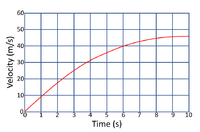# Terminal Velocity

## Key Stage 4

### Meaning

Terminal velocity is the velocity a falling object reaches when the forces of drag and weight on that object are balanced.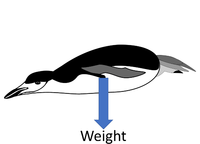At the start the penguin is not yet moving and the only force acting on the penguin is its weight. So the resultant force is large which means the acceleration will be large.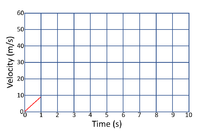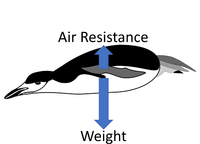As the penguin starts to accelerate its small velocity results in an air resistance that acts against its weight. So the resultant force is less than it was so the acceleration will be less.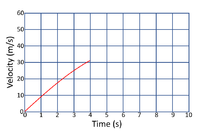As the penguin accelerates more its hight velocity results in more air resistance that acts against its weight. So the resultant force is even smaller than it was so the acceleration will be smaller.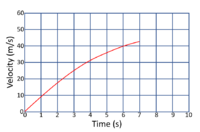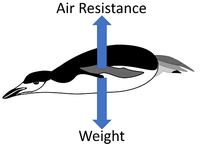Once the penguin is moving fast enough the velocity results in an air resistance that is as large as the weight. Resultant Force = 0N So there is no resultant force and so the acceleration will be zero. The penguin has reached terminal velocity.